# Different Combinations of Coins to Make an Amount

Different Combinations of Coins to Make an Amount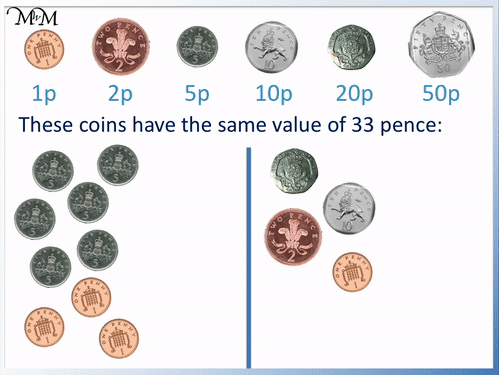• Both combinations of coins below have the same total value of 33 pence.
• The 20p coin is worth the same as four 5p coins.
• The 10p coin is worth the same as two 5p coins.
• The 2p coin is worth the same as two 1p coins.
• The single 1p coins shown are the same value.

We can add a combination of coins to make another coin.

Start by adding the largest value coins.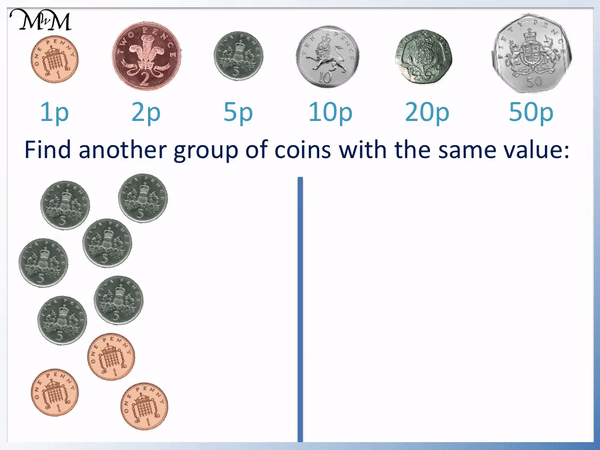• Here we have six 5p coins and three 1p coins.
• We will try to add the coins to make any of the coins listed at the top.
• We can add four 5p coins to make 20p.
• The remaining two 5p coins make 10p.
• The three 1p coins make 3p but there is no 3p coin.
• We can add two of the 1p coins to make 2p.
• Both combinations of coins add to make 33 pence.# How to Make an Amount using Different Combinations of Coins

To make a different combination of coins with the same value as another, group the coins into values that add to make other coins, starting with the largest value coins.

It helps to have a list of the other coins that can be chosen and to write down these values when you can make them.

Here is an example of finding an equivalent amount of money to the amount shown.

We have: two 10p coins, a 5p coin and two 1p coins.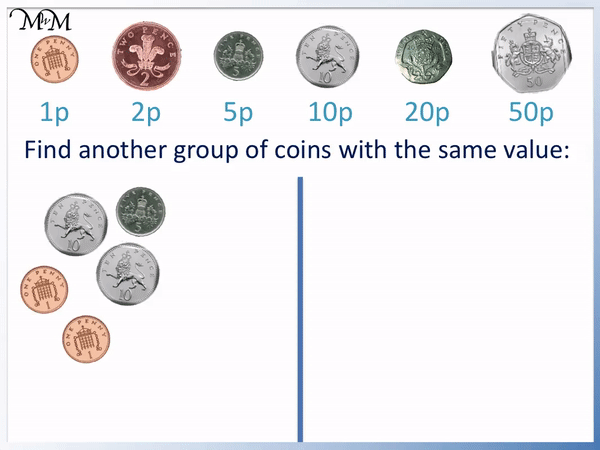We start by adding the coins with the largest value. We will add the two 10p coins.

Two 10p coins are worth 20p. There is a 20 pence coin and so we will write this down.

The 5p coin cannot be added to two 1p coins to make a new coin. This is because there is no 6p or 7p coin.

We keep the 5p coin as a 5p coin.

The remaining two 1p coins can be added to make 2p. There is a 2p coin which can be used instead of two 1p coins.

The total of the money is 27 pence.

27 pence can be made from the first combination of money: two 10p coins, one 5p coin and two 1p coins.

27 pence can also be made from the new combination of coins: one 20p coin, one 5p coin and one 2p coin.

It is possible to make this amount of money in many other ways. For example we could simply have twenty-seven 1p coins.

In this lesson we will be looking to add coins together to make the amount with the least amount of coins.

In this example we have the following collection of coins:

Six 5p coins and three 1p coins.We start by adding up the largest value coins. We will start by adding the 5p coins.

We cannot make 50p because six 5p coins added together makes 30p.

We will try and make 20p. This is the same as four 5p coins added together.

We write down 20p now that we have counted four of the six 5p coins.

There are two 5p coins left, which can make 10p.

The three 1p coins make 3p in total but there is not a 3 pence coin. We can use two of the 1p coins to make a 2p coin and then leave the final third 1 pence coin as a 1p.

The total of this amount of money is 33 pence.

33 pence can also be made from a 20p, a 10p, a 2p and a 1p coin.

The 20p is the same value as the four 5p coins, the 10p is the same value as the other two 5p coins and the 2p plus the 1p are the same as three 1p coins.

In this example, we have the following coins:

Two 20p coins, one 10p coin, two 2p coins and a 1p coin.

We will start by adding the largest value coins.

We can add two 20p coins to make 40 pence. However there is no 40p coin.

40p is only 10p less than 50p and we have a 10p coin.

20p plus 20p plus 10p is the same as 50p.

We can write down 50p.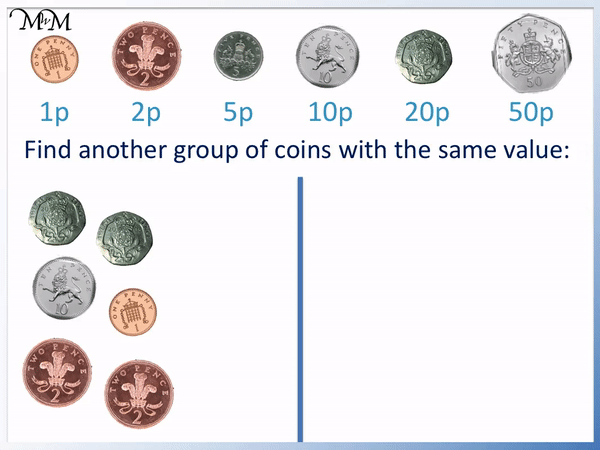We can add the two 2p coins to make 4p and again, there is no 4p coin. We can add the 1p coin to this amount to make 5p.

The two 2p coins plus the 1p coin can be replaced with a 5p coin.

The total of the money is 55 pence.

Instead of using the original combination of coins, we can also make 55p from a single 50p coin and a single 5p coin.

In this example we have three 10p coins, two 5p coins and three 2p coins.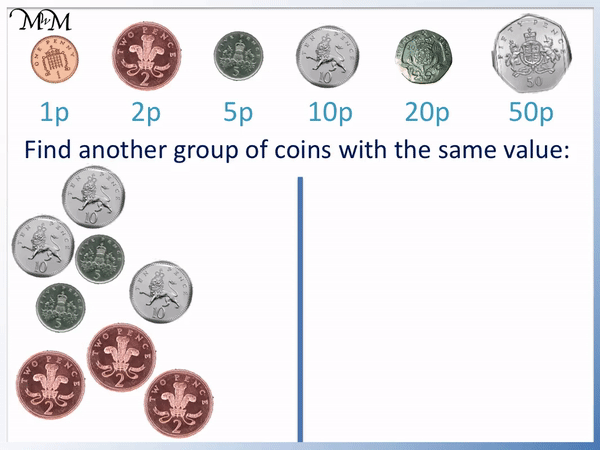We start by adding the largest value coins to try to make another coin.

If we add the three 10p coins, we have 30 pence. There is no 30 pence coin.

Even if we add the two 5p coins to this amount, we would only have 40 pence. There is no 40 pence coin. Because we cannot make the 50p coin with this money, we will try to make the next value coin, 20p.

Instead, two 10p coins can be used to make 20p.

The remaining 10p plus the two 5p coins can be used to make another 20p coin.

The three 2p coins add to make 6 pence. There is no 6p coin.

Instead this value of 6p can be made from a 5p coin plus a 1p coin.

The amount of 46 pence can be made from two 20p coins, one 5p coin and one 1p coin.Now try our lesson on Counting US Coins: Dimes, Nickels, Pennies & Quarters where we learn the values of American coins.error: Content is protected !!